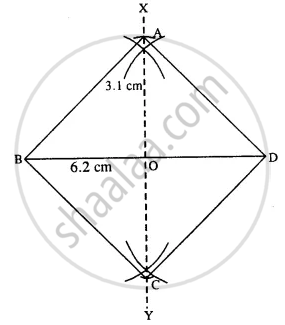# Construct a Square, If : One Diagonal is 6.2 Cm. - Mathematics

Sum

Construct a square, if :

one diagonal is 6.2 cm.

#### SolutionSteps :

1. Draw BD = 6.2 cm.
2. Draw perpendicular bisector XY of BD.
3. Cut OA = OC = 3.1 cm (half the diagonal)
4. Join AB, AD, BC, and CD.
Thus ABCD is the required square.
Concept: Construction of Square
Is there an error in this question or solution?

#### APPEARS IN

Selina Concise Mathematics Class 8 ICSE
Chapter 18 Constructions
Exercise 18 (D) | Q 5.3 | Page 211